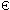Next: Karp-Rabin algorithm Up: ESMAJ Prev: Brute Force algorithm

# Research with an automaton

Main features
• builds the minimal deterministic automaton recognizing the language*x;
• extra space in O(m) if the automaton is stored in a direct access table;
• preprocessing phase in O(m) time complexity;
• searching phase in O(n) time complexity if the automaton is stored in a direct access table, O(nlog()) otherwise.
Description

Searching a word x with an automaton consists first in building the minimal Deterministic Finite Automaton (DFA)  A(x) recognizing the language*x.

The DFA  A(x) =(Q, q0, T, E) recognizing the language*x is defined as follows:Q is the set of all the prefixes of x: Q={, x, x[0 .. 1], ... , x[0 .. m-2], x};q0=;T={x};for q in Q (q is a prefix of x) and a in, (q, a, qa) is in E if and only if qa is also a prefix of x, otherwise (q, a, p) is in E such that p is the longest suffix of qa which is a prefix of x.

The DFA  A(x) can be constructed in O(m+) time and O(m) space.

Once the DFA  A(x) is build, searching for a word x in a text y consists in parsing the text y with the DFA  A(x) beginning with the initial state q0. Each time the terminal state is encountered an occurrence of x is reported.

The searching phase can be performed in O(n) time if the automaton is stored in a direct access table, in O(nlog()) otherwise.

The C code
```void preAut(char *x, int m, Graph aut) {
int i, state, target, oldTarget;

for (state = getInitial(aut), i = 0; i < m; ++i) {
oldTarget = getTarget(aut, state, x[i]);
target = newVertex(aut);
setTarget(aut, state, x[i], target);
copyVertex(aut, target, oldTarget);
state = target;
}
setTerminal(aut, state);
}

void AUT(char *x, int m, char *y, int n) {
int j, state;
Graph aut;

/* Preprocessing */
aut = newAutomaton(m + 1, (m + 1)*ASIZE);
preAut(x, m, aut);

/* Searching */
for (state = getInitial(aut), j = 0; j < n; ++j) {
state = getTarget(aut, state, y[j]);
if (isTerminal(aut, state))
OUTPUT(j - m + 1);
}
}

```
The example

Preprocessing phaseThe states are labelled by the length of the prefix they are associated with.
Missing transitions are leading to the initial state 0.

Searching phase

References
• CORMEN, T.H., LEISERSON, C.E., RIVEST, R.L., 1990. Introduction to Algorithms, Chapter 34, pp 853-885, MIT Press.
• CROCHEMORE, M., 1997. Off-line serial exact string searching, in Pattern Matching Algorithms, ed. A. Apostolico and Z. Galil, Chapter 1, pp 1-53, Oxford University Press.
• CROCHEMORE, M., HANCART, C., 1997. Automata for Matching Patterns, in Handbook of Formal Languages, Volume 2, Linear Modeling: Background and Application, G. Rozenberg and A. Salomaa ed., Chapter 9, pp 399-462, Springer-Verlag, Berlin.
• GONNET, G.H., BAEZA-YATES, R.A., 1991. Handbook of Algorithms and Data Structures in Pascal and C, 2nd Edition, Chapter 7, pp. 251-288, Addison-Wesley Publishing Company.
• HANCART, C., 1993. Analyse exacte et en moyenne d'algorithmes de recherche d'un motif dans un texte, Ph. D. Thesis, University Paris 7, France.Next: Karp-Rabin algorithm Up: ESMAJ Prev: Brute Force algorithm

e-mails: {Christian.Charras, Thierry.Lecroq} @laposte.net
Tue Jan 14 15:03:31 MET 1997